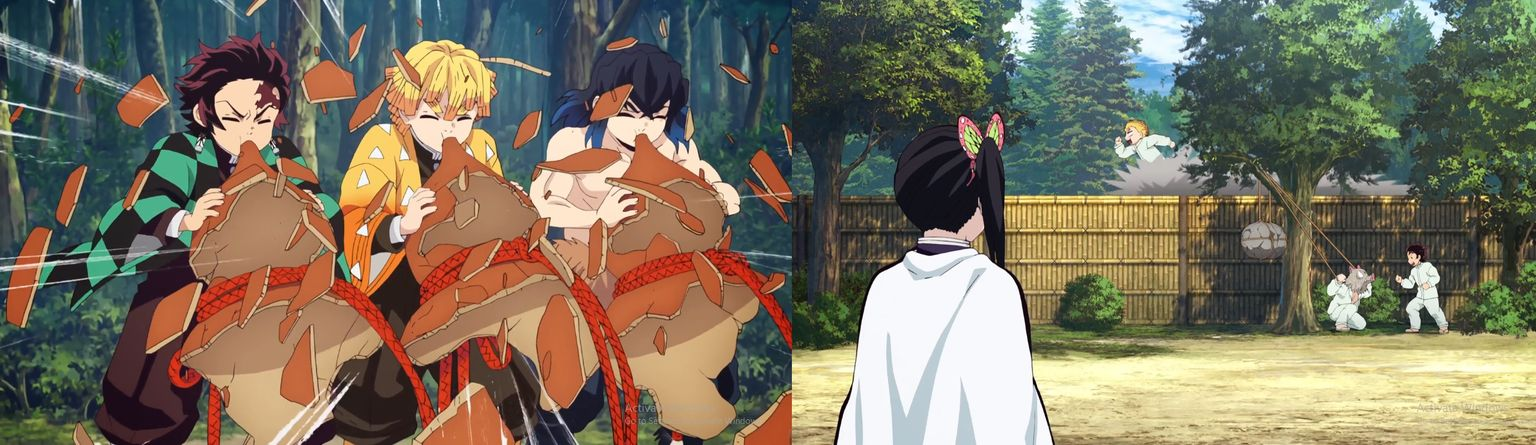# Practice on Toph

Participate in exhilarating programming contests, solve unique algorithm and data structure challenges and be a part of an awesome community.

# Here Is a Treat for You 🍕🍔

By tanimahossain · Limits 1s, 512 MB

Kagaya Ubuyashiki, the 97-th leader of the Demon Slayer Corps, Also known as Oyakata-sama to the Demon Slayer Corps, just met Tanjiro, Zenitsu, and Inosuke. Instantly, he knew there is something special about them. But as special they are, they also need special training to make their ability grow faster as well. You know they can be trained best by the hashiras(highest rank of demon slayers) but the hashiras are busy slaying demons (Don’t worry they respect the pandemic they are wearing masks, and saving people from demons is more important to them than quarantining right now). They are spread all around the world. They can’t come to the demon slayer headquarters to teach them. So, the Oyakata-Sama made a scheme so that they can learn properly.

Initially, Tanjiro, Zenitsu, and Inosuke each knew only one breathing technique. Then all three meet at the demon slayer headquarters every time they get free to teach their breathing techniques to each other. After that, they go separate ways and practice those breathing techniques under different hashiras. Tanjiro practices the techniques shown by Zenitsu for $x_1$ days and after that, Inosuke’s for $y_1$ days. But, as Tanjiro goes to train under the hashiras, they influence those techniques in a way that the number of breathing techniques Tanjiro knows increases by the summation of $x_1$ × The number of techniques Zenitsu showed and $y_1$ × The number of techniques Inosuke showed. In the same way, Zenitsu’s and Inosuke’s practices get influenced following the rule mentioned below-

Lets say, after i-th meeting and finishing practice the number of breathing techniques Tanjiro, Zanitsu and Inosuke know are $T_{i}$, $Z_{i}$ and $I_{i}$ respectively. So,

$T_{i}$ = $T_{i-1}$ + ( $Z_{i-1}$ × $x_1$ ) + ( $I_{i-1}$ × $y_1$ )

$Z_{i}$ = $Z_{i-1}$ + ( $T_{i-1}$ × $x_2$ ) + ( $I_{i-1}$ × $y_2$ )

$I_{i}$ = $I_{i-1}$ + ( $T_{i-1}$ × $x_3$ ) + ( $Z_{i-1}$ × $y_3$ )Today is such a day! They are going to meet again and Oyakata-Sama told me that they have met $N$ times before today. So, I was wondering how many techniques Tanjiro know before the meeting (Why Tanjiro? I’ll leave it to your imagination 😉). Anyway, the number of techniques Tanjiro know at this point might be so big that it might not fit into a 32-bit integer ( this is Demon Slayer in some parallel universe anything is possible 😉) that is why you have to output the value modulo $10^{9}+7(1000000007)$. In other words, Print $T_n\%1000000007$, initially $T_0=1, Z_0=1, I_0=1$.

## Input

The first line will be a single integer $T$ indicating the number of test cases. The following $T$ lines will contain seven integers $x_1$, $y_1$, $x_2$, $y_2$, $x_3$, $y_3$, $N$ separated by spaces.

Constraints:

$1 ≤ T ≤ 10^{3}$

$0 ≤ x_1, y_1, x_2, y_2, x_3, y_3 ≤ 10^{9}$

$1 ≤ N ≤ 10^{9}$

## Output

You have to output $T$ lines in format “$Case X: Y$”(without quotes) where $X$ is the number of test case and $Y$ is $T_n\%1000000007$.

## Sample

InputOutput
3
1 2 0 0 0 0 3
1 1 1 1 1 1 4
1 0 1 0 1 0 10

Case 1: 10
Case 2: 81
Case 3: 1024


For the 1st test case Initially, Tanjiro knows 1 technique, Zenitsu knows, 1 technique Inosuke knows 1 technique

After 1st meeting and finishing their practice,

Tanjiro knows 1+1×1+2×1=4 techniques Zenitsu knows 1+0×1+0×1=1 technique Inosuke knows 1+0×1+0×1=1 technique

After 2nd meeting and finishing their practice,

Tanjiro knows 4+1×1+2×1=7 techniques Zenitsu knows 1+0×1+0×1=1 technique Inosuke knows 1+0×1+0×1=1 technique

After 3rd meeting and finishing their practice, Tanjiro, Zenitsu and Inosuke know 10 techniques, 1 technique and 1 technique respectively.

### Statistics

75% Solution Ratio

alamkhanEarliest, 3d ago

tanimahossainFastest, 0.0s

alamkhanLightest, 131 kB

steinumShortest, 1028B

### Submit

Login to submit

### Editorial

Observation: Number of techniques for each of them depends on the number of techniques they had in t...

### Related Contests

 DIU Take-Off Programming Contest Spring 2020Ended 1w ago